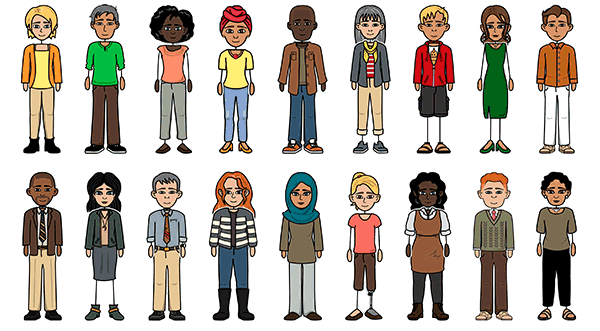# COMIC STRIP ABOUT ARITHMETIC SEQUENCE

Create a Storyboard#### Storyboard Description

Submitted by: Mara Grace D. Mendez 10-Faith

#### Storyboard Text

• Yey that's great!! When will we meet?
• Yes ofcourse.
• Actually i'm not doing fine. We're learning about Arithmetic Sequences in math and i'm so confused!!
• Oh! I have a friend who can teach you.
• Hi Melissa how are you?
• How about later? I'll just text you is that okay?﻿
• I don't know how to determine if a sequence is an arithmetic sequence let alone how to determinethe pattern of one.
• The common difference? What does that mean?
• Ok, well you need to find the common difference of the pattern.
• 4,6,8,10
• a , a .... 1 2
• d = 6-4 =2d = 8-6 =2d = 10-8 =2
• d= Common differenced= a -a 2 1
• it is an arithmetic sequence.
• The common difference is the pattern between the number in an arithmetic sequence. So, if we have a sequence of numbers such as 4,6,8,10 we'd take the 2nd number and subtact the 1st number.
• 4,6,8,10
• a , a .... 1 2
• so then, how would we determine if isn't an arithmetic sequence
• The common difference would not be the same throughout. The common difference has to be the same for it to ba an arithmetic sequence.
• d = 6-4 =2d = 8-6 =2d = 10-8 =2
• d= Common differenced= a -a 2 1
• it is an arithmetic sequence.
• So the common difference of this of this sequence number would be 2 because of the common difference is the same throughout.
• 4,7,10,14
• Oh ok!
• Well, the common difference is 3 between the first two sets of numbers but not the third, so it isn't an arithmetic sequence
• d = 7-4 =3d = 10-7 =3d = 14-10 =4
• Here you try.Your sequence of numbers are 4,7,10,14
• It is NOT an arithmetic sequence.
• 4,7,10,14
• Oh ok, that's not bad! They're pretty fun actually!!
• d = 7-4 =3d = 10-7 =3d = 14-10 =4
• Exactly! See you got it!!
• It is NOT an arithmetic sequence.
Over 30 Million Storyboards Created